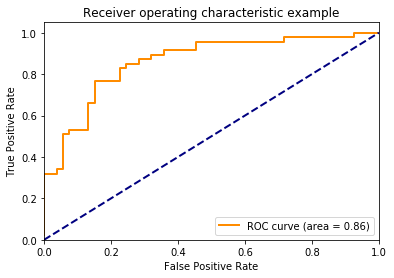# Evaluating a Machine Learning Model Part 2¶

### Elan Ding¶

Modified: June 27, 2018

We are going to talk about the receiver operating characteristic (ROC) curve. The ROC curve is a commonly used tool to evaluate the performance of a binary classifier as its discriminant threshhold varies. What does it mean by discriminant threshhold? Allow me to explain.

For a classification task we can always set

$$Y = \begin{cases} 0 & \text{ if negative} \\ 1 & \text{ if positive} \end{cases}$$

Now that $Y$ becomes quantitative, we can be clever and fit a linear regression to this. Intuitively, we can classify $Y$ as negative if $\widehat{Y}<0.5$ and positive otherwise. Indeed when $Y$ is binary, this technique is equivalent to linear discriminant analysis.

Since $Y$ is binary, the regression function can be interpreted as a probability: $E(Y\,|\,X=x) = P(Y=1\,|\, X=x)$. (Note that for linear discriminant analysis, this is not strickly a probaility since it can be negative or greater than 1. Nevertheless, it is a confidence measure.) This probability tells us how confident we are about classifying $Y$ as positive. How confident do we need to be in order to classify $Y$ as positive? This confidence is the threshhold value.

In my previous post, I showed that the decision boundary for the logistic regression is

$$\beta_0 + \boldsymbol{\beta}^{\text{T}}\boldsymbol{X} = \ln k$$

Here the threshhold is the value of $\ln k$ or $k$.

## The ROC Curve¶

Here is an example of an ROC curve:The ROC curve plots the true positive rate (TP) against the false positive rate (FP) as the threshhold value varies in a binary classifier. In other words, it plots type I error $\alpha$ against specificity $1-\beta$ as we shift the confidence of classification.

The dashed diagonal line in the plot refers to the ROC curve of a random model. This means that regardless of the data, we will just flip a coin: If the coin lands heads, we will classify $Y$ as positive, and $0$ otherwise. Suppose we are flipping a biased coin with tails on both sides. We are never getting heads, so the sensitivity has to be 0, and so is the true positive rate. We are at the bottom left corner.

In general, flipping a coin means that we are ignoring the true value of $Y$. Hence the false positive rate must equal to the true positive rate (the "false" and "true" is irrelavant to a coin flip). This is why we have a diagonal line in the ROC plot.

We can test this out in Python.

In :
import numpy as np
import matplotlib.pyplot as plt
from sklearn.metrics import confusion_matrix
%matplotlib inline


Next we are going to generate the output variable as a binary vector of 0 and 1's.

In :
np.random.seed(111)
truth = np.random.randint(0,2,1000)


Define a coin flip function that has various probability of rolling heads:

In :
def coin_flip(prob):
return 1 if np.random.random() < prob else 0


We flip a fair coin to classify the output, and we plot false positive against true positive value.

In :
prediction = [coin_flip(0.5) for i in range(1000)]
cm = confusion_matrix(truth, prediction).transpose()
FP = cm[1,0]/(cm[1,0]+cm[0,0])
TP = cm[1,1]/(cm[1,1]+cm[0,1])

In :
plt.figure()
plt.plot([FP], [TP], marker='o', markersize=8, color = 'b')
plt.xlabel('False Positive')
plt.ylabel('True Positive')
plt.xlim([0.0, 1.0])
plt.ylim([0.0, 1.0])
plt.show()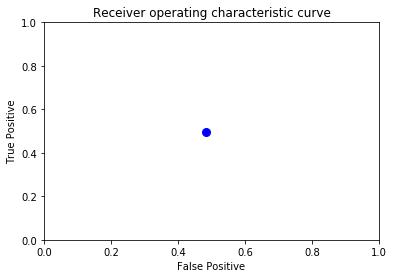Note that the dot lands rougly around $(0.5, 0.5)$. This is to be expected since our original data was also randomly generated, so by randomness, we have rougly 50% probability of making both true and false positive claims. Next we plot the TP-FP pairs for various threshhold values:

In :
plt.subplots()

for prob in np.arange(0, 1.1, 0.1):
cm = confusion_matrix(truth, prediction).transpose()
FP = cm[1,0]/(cm[1,0]+cm[0,0])
TP = cm[1,1]/(cm[1,1]+cm[0,1])
prediction = [coin_flip(prob) for i in range(1000)]
plt.plot([FP], [TP], marker='o', markersize=8, color = 'b')

plt.xlabel('False Positive')
plt.ylabel('True Positive')
plt.xlim([0.0, 1.0])
plt.ylim([0.0, 1.0])
plt.show()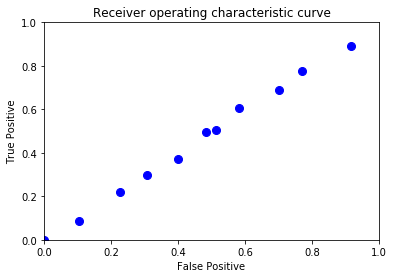Indeed they lie on the diagonal. So what about the orange curve? For a random classifier, we are most likely never going to obtain a ROC curve that is significantly above the diagonal line. Of course, we want the ROC curve to reach the upper left region, where the model has the highest true positive rate and lowest false positive rate. This also suggest that the larger it is the area under the ROC curve (AUC), the better the classifier performs.

## Example 1¶

First we give a very simple example.

In :
from sklearn.metrics import roc_curve, auc


Suppose that the true values of binary random variable are $(0, 0, 1, 1)$, and the scores, or how confident we are that each one is equal to 1, are $(0.1, 0.4, 0.35, 0.8)$. Enter them into the roc_curve function:

In :
y = np.array([0, 0, 1, 1])
scores = np.array([0.1, 0.4, 0.35, 0.8])
fpr, tpr, threholds = roc_curve(y, scores)
roc_auc = auc(fpr, tpr)

In :
print(fpr, tpr, threholds, roc_auc)

[0.  0.5 0.5 1. ] [0.5 0.5 1.  1. ] [0.8  0.4  0.35 0.1 ] 0.75


Note that we have four different threholds levels $(0.8, 0.4, 0.35, 0.1)$. For example, suppose we use the smallest threshhold value of $0.1$. This means that all four variables are going to be classified as 1. In this case, the true positive rate is 1 because our prediction correctly classified both 1's. However, the false positive rate is also 1 because both 0's are incorrectly classified. The model performs the best when the threshold value is at 0.8, which gives a false positive rate of 0 and true positive rate 0.5. Let's visualize this.

In :
plt.figure()
lw = 2
plt.plot(fpr, tpr, color='darkorange',
lw=lw, label='ROC curve (area = %0.2f)' % roc_auc)
plt.plot([0, 1], [0, 1], color='navy', lw=lw, linestyle='--')
plt.xlim([0, 1.0])
plt.ylim([0, 1.05])
plt.xlabel('False Positive Rate')
plt.ylabel('True Positive Rate')
plt.legend(loc="lower right")
plt.show()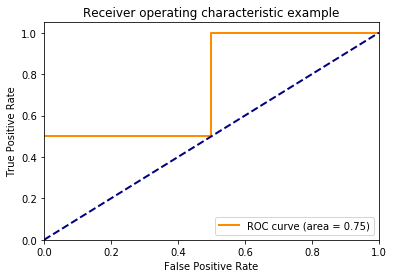## Example 2¶

In :
from sklearn.datasets import make_blobs
from sklearn.model_selection import train_test_split
from sklearn.linear_model import LogisticRegression


We generate a data set of 200 points, 2 features, and 2 clusters with a small standard deviation in each cluster, so that we can see a clear separation of classes in the plane.

In :
X, y = make_blobs(n_samples=200, n_features=2, centers=2, cluster_std=2, random_state=101)


Pull out half of our data set as the test set.

In :
X_train, X_test, y_train, y_test = train_test_split(X, y, test_size=.5)


We fit a logistic regression model. Note that decision_function is used to calculate the score of our predictions. According to the documentation it is computed as the distance of the data point to the decision boundary of the model.

In :
logmodel = LogisticRegression()
logmodel.fit(X_train, y_train)

Out:
LogisticRegression(C=1.0, class_weight=None, dual=False, fit_intercept=True,
intercept_scaling=1, max_iter=100, multi_class='ovr', n_jobs=1,
penalty='l2', random_state=None, solver='liblinear', tol=0.0001,
verbose=0, warm_start=False)
In :
logmodel.coef_

Out:
array([-0.42326939, -0.79033051])
In :
logmodel.intercept_

Out:
array([-2.30065262])
In :
plt.scatter(X[:,0], X[:,1], c=y, cmap='rainbow')
a = np.linspace(-15, 5, 100)
b = -(logmodel.coef_[0,0]/logmodel.coef_[0,1])*a - logmodel.intercept_/logmodel.coef_[0,1]
plt.plot(a,b, c='y', linewidth=4)

Out:
[<matplotlib.lines.Line2D at 0x7f184f54ef28>]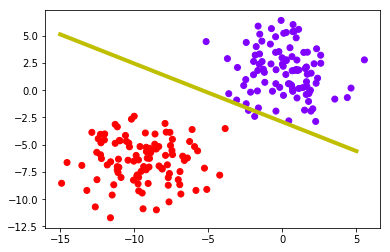Indeed, there is a wide separation between the two classes, so we expect the linear model to perform very well. We check the scores next, which is the distance between each data point to the decision boundary.

In :
y_score = logmodel.decision_function(X_test)
plt.hist(y_score)

Out:
(array([ 7., 20., 11.,  9.,  2.,  5., 15., 19.,  7.,  5.]),
array([-7.32443028, -5.40574218, -3.48705407, -1.56836596,  0.35032214,
2.26901025,  4.18769835,  6.10638646,  8.02507457,  9.94376267,
11.86245078]),
<a list of 10 Patch objects>)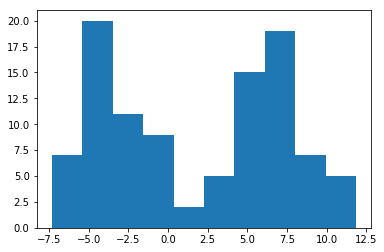The bimodal shape is expected. Next we plot the ROC curve below.

In :
fpr, tpr, _ = roc_curve(y_test, y_score)
roc_auc = auc(fpr, tpr)

In :
plt.figure()
lw = 2
plt.plot(fpr, tpr, color='darkorange',
lw=lw, label='ROC curve (area = %0.2f)' % roc_auc)
plt.plot([0, 1], [0, 1], color='navy', lw=lw, linestyle='--')
plt.xlim([0.0, 1.0])
plt.ylim([0.0, 1.05])
plt.xlabel('False Positive Rate')
plt.ylabel('True Positive Rate')
plt.legend(loc="lower right")
plt.show()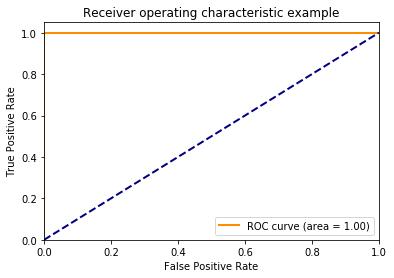Well, that's not very interesting! It is actually the BEST case scenario. It is the ROC curve of a perfect model. In reality this never happens! So let's increase the variance of the data:

In :
X, y = make_blobs(n_samples=200, n_features=2, centers=2, cluster_std=8, random_state=101)
X_train, X_test, y_train, y_test = train_test_split(X, y, test_size=.5)
logmodel = LogisticRegression()
y_score = logmodel.fit(X_train, y_train).decision_function(X_test)

plt.scatter(X[:,0], X[:,1], c=y, cmap='rainbow')
a = np.linspace(-20, 10, 100)
b = -(logmodel.coef_[0,0]/logmodel.coef_[0,1])*a - logmodel.intercept_/logmodel.coef_[0,1]
plt.plot(a,b, c='y', linewidth=4)

Out:
[<matplotlib.lines.Line2D at 0x7f184f448cf8>]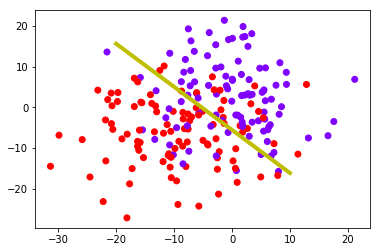In :
fpr, tpr, _ = roc_curve(y_test, y_score)
roc_auc = auc(fpr, tpr)

plt.figure()
lw = 2
plt.plot(fpr, tpr, color='darkorange',
lw=lw, label='ROC curve (area = %0.2f)' % roc_auc)
plt.plot([0, 1], [0, 1], color='navy', lw=lw, linestyle='--')
plt.xlim([0.0, 1.0])
plt.ylim([0.0, 1.05])
plt.xlabel('False Positive Rate')
plt.ylabel('True Positive Rate')